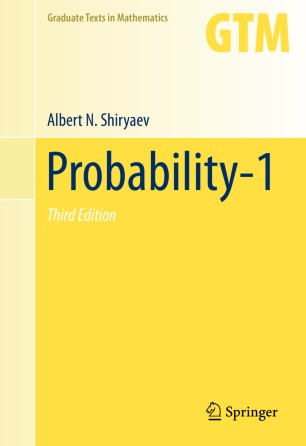# Probability-1

## Volume 1

• Albert N. ShiryaevTextbook

Part of the Graduate Texts in Mathematics book series (GTM, volume 95)

1. Front Matter
Pages i-xvii
2. Albert N. Shiryaev
Pages 1-158
3. Albert N. Shiryaev
Pages 159-371
4. Albert N. Shiryaev
Pages 373-460
5. Back Matter
Pages 461-486

### Introduction

This book contains a systematic treatment of probability from the ground up, starting with intuitive ideas and gradually developing more sophisticated subjects, such as random walks, martingales, Markov chains, the measure-theoretic foundations of probability theory, weak convergence of probability measures, and the central limit theorem. Many examples are discussed in detail, and there are a large number of exercises. The book is accessible to advanced undergraduates and can be used as a text for independent study.

To accommodate the greatly expanded material in the third edition of Probability, the book is now divided into two volumes. This first volume contains updated references and substantial revisions of the first three chapters of the second edition. In particular, new material has been added on generating functions, the inclusion-exclusion principle, theorems on monotonic classes (relying on a detailed treatment of “π-λ” systems), and the fundamental theorems of mathematical statistics.

### Keywords

Ergodic theory Markov chain Martingale Probability theory Random variable Random walk Stochastic process Stochastic processes

#### Authors and affiliations

• Albert N. Shiryaev
• 1
1. 1.Department of Probability Theory and Mathematical StatisticsSteklov Mathematical Institute and Lomonosov Moscow State UniversityMoscowRussia